# 【Unity3D开发小游戏】扫雷

@[TOC]

## 二、源码

UI资源和源代码请搜索QQ群：1040082875下载

## 三、正文

Unity 2D 扫雷游戏教程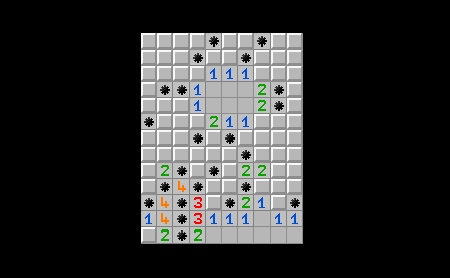### 项目设置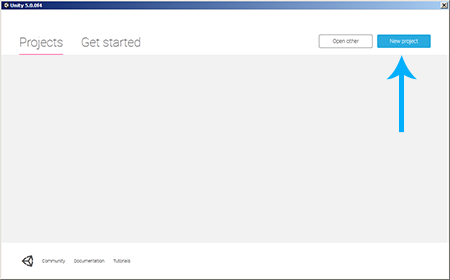我们将该项目命名为minesweeper，选取项目存储的目录如C:\，项目类型选择2D，然后点击Create Project：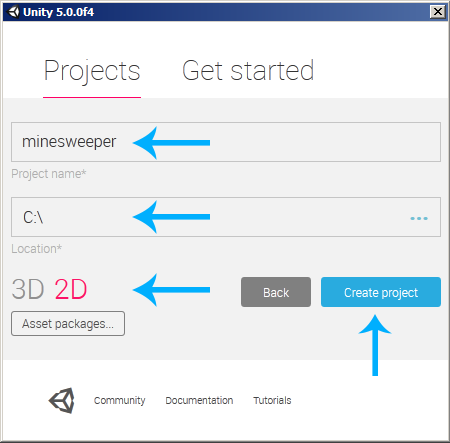在层次面板（Hierarchy）中选中Main Camera，将背景色（Background Color）设置为黑色。并如下图所示调整大小和位置：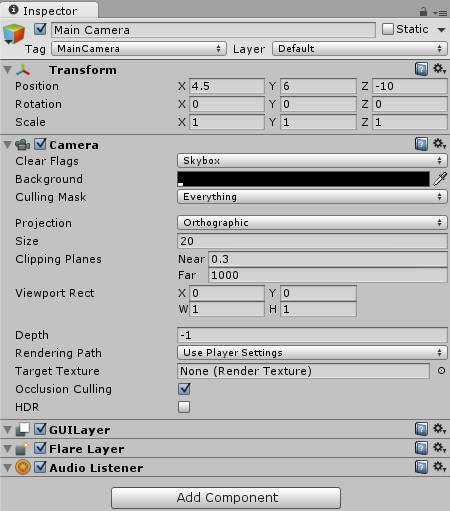### 默认方块

Colliders是物理对象的一部分。现在，我们的方块在游戏世界里就是一张图片，我们为它添加一个Colliders组件，该方块将成为物理世界的一部分咯，就像一堵墙。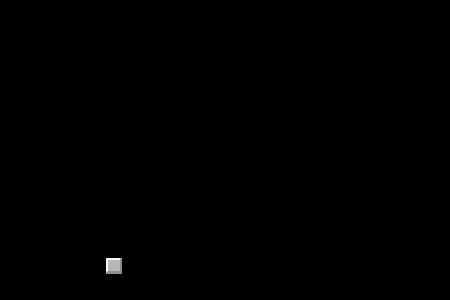### 关于邻接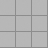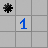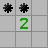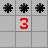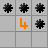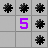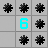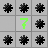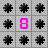因此，我们所要做的就是计算每个字段的相邻地雷数量，然后得出数字，如果没有相邻的地雷，则什么也不画。

### Element.cs：

``````using UnityEngine;
using System.Collections;

public class Element : MonoBehaviour {

// Use this for initialization
void Start () {

}

// Update is called once per frame
void Update () {

}
}
``````

``````using UnityEngine;
using System.Collections;

public class Element : MonoBehaviour {

// Is this a mine?
public bool mine;

// Use this for initialization
void Start () {

}
}
``````

``````using UnityEngine;
using System.Collections;

public class Element : MonoBehaviour {

// Is this a mine?
public bool mine;

// Use this for initialization
void Start () {
// Randomly decide if it's a mine or not
mine = Random.value < 0.15;
}
}
``````

``````using UnityEngine;
using System.Collections;

public class Element : MonoBehaviour {

// Is this a mine?
public bool mine;

// Different Textures
public Sprite[] emptyTextures;
public Sprite mineTexture;

// Use this for initialization
void Start () {
// Randomly decide if it's a mine or not
mine = Random.value < 0.15;
}
}
``````

``````using UnityEngine;
using System.Collections;

public class Element : MonoBehaviour {

// Is this a mine?
public bool mine;

// Different Textures
public Sprite[] emptyTextures;
public Sprite mineTexture;

// Use this for initialization
void Start () {
// Randomly decide if it's a mine or not
mine = Random.value < 0.15;
}

if (mine)
GetComponent<SpriteRenderer>().sprite = mineTexture;
else
}
}
``````

*注意：函数首先检查元素是否是地雷。如果它是一个地雷，那么它加载地雷的纹理。如果它不是地雷，它就会装载一个空的Texture，取决于邻接计数..这个GetComponent().sprite问题是我们如何改变当前的纹理。*

``````// Use this for initialization
void Start () {
// Randomly decide if it's a mine or not
//mine = Random.value < 0.15;

// TEST
}
``````

``````// Use this for initialization
void Start () {
// Randomly decide if it's a mine or not
mine = Random.value < 0.15;
}
``````

``````// Is it still covered?
public bool isCovered() {
return GetComponent<SpriteRenderer>().sprite.texture.name == "default";
}
``````

``````void OnMouseUpAsButton() {
// ToDo: do stuff..
}
``````

``````void OnMouseUpAsButton() {
// It's a mine
if (mine) {
// ToDo: do stuff..
}
// It's not a mine
else {
// ToDo: do stuff..
}
}
``````

``````void OnMouseUpAsButton() {
// It's a mine
if (mine) {
// ToDo: uncover all mines
// ...

// game over
print("you lose");
}
// It's not a mine
else {
// ToDo: do stuff..
}
}
``````

``````void OnMouseUpAsButton() {
// It's a mine
if (mine) {
// ToDo: uncover all mines
// ...

// game over
print("you lose");
}
// It's not a mine
else {
// ToDo show adjacent mine number

// ToDo uncover area without mines
// ...

// ToDo find out if the game was won now
// ...
}
}
``````

### Grid

``````using UnityEngine;
using System.Collections;

public class Grid : MonoBehaviour {

// Use this for initialization
void Start () {

}

// Update is called once per frame
void Update () {

}
}
``````

``````using UnityEngine;
using System.Collections;

public class Grid {

}
``````

### 元素二维阵列

``````using UnityEngine;
using System.Collections;

public class Grid {
// The Grid itself
public static int w = 10; // this is the width
public static int h = 13; // this is the height
public static Element[,] elements = new Element[w, h];
}
``````

### 初始化网格

``````// Use this for initialization
void Start () {
// Randomly decide if it's a mine or not
mine = Random.value < 0.15;

// Register in Grid
int x = (int)transform.position.x;
int y = (int)transform.position.y;
Grid.elements[x, y] = this;
}
``````

### 发现所有地雷

``````// Uncover all Mines
public static void uncoverMines() {
foreach (Element elem in elements)
if (elem.mine)
}
``````

``````void OnMouseUpAsButton() {
// It's a mine
if (mine) {
// Uncover all mines
Grid.uncoverMines();

// game over
print("you lose");
}
// It's not a mine
else {
// ToDo show adjacent mine number

// ToDo uncover area without mines
// ...

// ToDo find out if the game was won now
// ...
}
}
``````

• 右上角
• 右（边），正确的
• 右下角
• 底部
• 左下角
• 左边
• 左上角 当其中一个元素是地雷时，增加一个计数器。

``````// Find out if a mine is at the coordinates
public static bool mineAt(int x, int y) {
// Coordinates in range? Then check for mine.
if (x >= 0 && y >= 0 && x < w && y < h)
return elements[x, y].mine;
return false;
}
``````

``````// Count adjacent mines for an element
public static int adjacentMines(int x, int y) {
int count = 0;

// ...

return count;
}
``````

``````// Count adjacent mines for an element
public static int adjacentMines(int x, int y) {
int count = 0;

if (mineAt(x,   y+1)) ++count; // top
if (mineAt(x+1, y+1)) ++count; // top-right
if (mineAt(x+1, y  )) ++count; // right
if (mineAt(x+1, y-1)) ++count; // bottom-right
if (mineAt(x,   y-1)) ++count; // bottom
if (mineAt(x-1, y-1)) ++count; // bottom-left
if (mineAt(x-1, y  )) ++count; // left
if (mineAt(x-1, y+1)) ++count; // top-left

return count;
}
``````

``````void OnMouseUpAsButton() {
// It's a mine
if (mine) {
// uncover all mines
Grid.uncoverMines();

// game over
print("you lose");
}
// It's not a mine
else {
int x = (int)transform.position.x;
int y = (int)transform.position.y;

// ToDo uncover area without mines
// ...

// ToDo find out if the game was won now
// ...
}
}
``````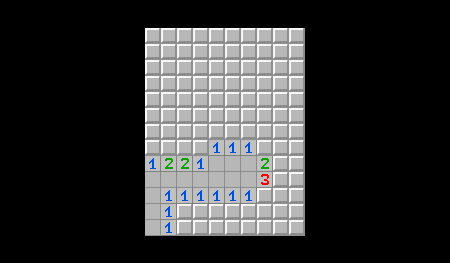### 发现一个区域

• 从某种元素开始
• 用这个元素做我们想做的事
• 对每个相邻元素递归地继续

``````// Flood Fill empty elements
public static void FFuncover(int x, int y, bool[,] visited) {
if (visited[x, y])
return;

// set visited flag
visited[x, y] = true;

// recursion
FFuncover(x-1, y, visited);
FFuncover(x+1, y, visited);
FFuncover(x, y-1, visited);
FFuncover(x, y+1, visited);
}
``````

``````// Flood Fill empty elements
public static void FFuncover(int x, int y, bool[,] visited) {
// Coordinates in Range?
if (x >= 0 && y >= 0 && x < w && y < h) {
if (visited[x, y])
return;

// set visited flag
visited[x, y] = true;

// recursion
FFuncover(x-1, y, visited);
FFuncover(x+1, y, visited);
FFuncover(x, y-1, visited);
FFuncover(x, y+1, visited);
}
}
``````

``````// Flood Fill empty elements
public static void FFuncover(int x, int y, bool[,] visited) {
// Coordinates in Range?
if (x >= 0 && y >= 0 && x < w && y < h) {
if (visited[x, y])
return;

// uncover element

// close to a mine? then no more work needed here
return;

// set visited flag
visited[x, y] = true;

// recursion
FFuncover(x-1, y, visited);
FFuncover(x+1, y, visited);
FFuncover(x, y-1, visited);
FFuncover(x, y+1, visited);
}
}
``````

``````void OnMouseUpAsButton() {
// It's a mine
if (mine) {
// uncover all mines
Grid.uncoverMines();

// game over
print("you lose");
}
// It's not a mine
else {
int x = (int)transform.position.x;
int y = (int)transform.position.y;

// uncover area without mines
Grid.FFuncover(x, y, new bool[Grid.w, Grid.h]);

// ToDo find out if the game was won now
// ...
}
}
``````

### 检查是否发现所有地雷

``````public static bool isFinished() {
// Try to find a covered element that is no mine
foreach (Element elem in elements)
if (elem.isCovered() && !elem.mine)
return false;
// There are none => all are mines => game won.
return true;
}
``````

``````void OnMouseUpAsButton() {
// It's a mine
if (mine) {
// uncover all mines
Grid.uncoverMines();

// game over
print("you lose");
}
// It's not a mine
else {
int x = (int)transform.position.x;
int y = (int)transform.position.y;

// uncover area without mines
Grid.FFuncover(x, y, new bool[Grid.w, Grid.h]);

// find out if the game was won now
if (Grid.isFinished())
print("you win");
}
}
``````

• 用标志标记元素
• 添加更大的级别
• 更漂亮的图形
• 一些不错的音效
• 输赢屏幕
• 重新启动按钮